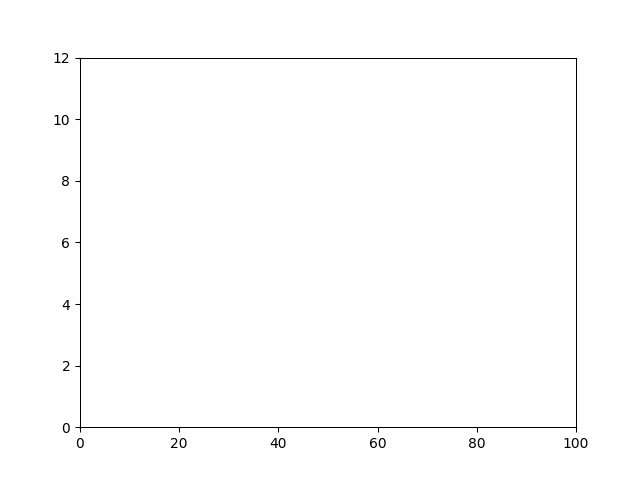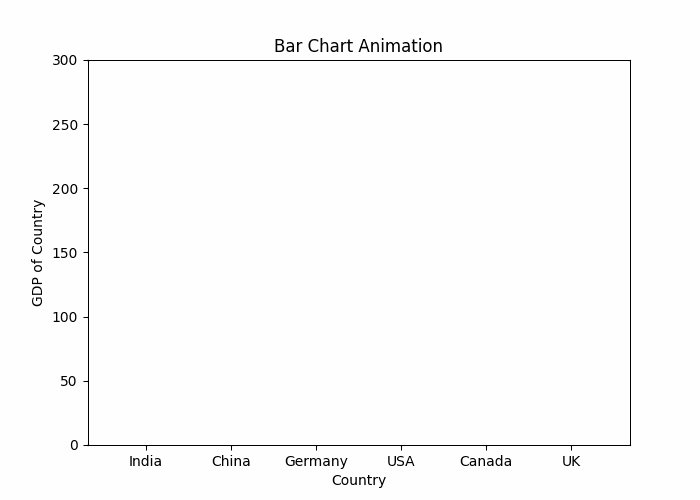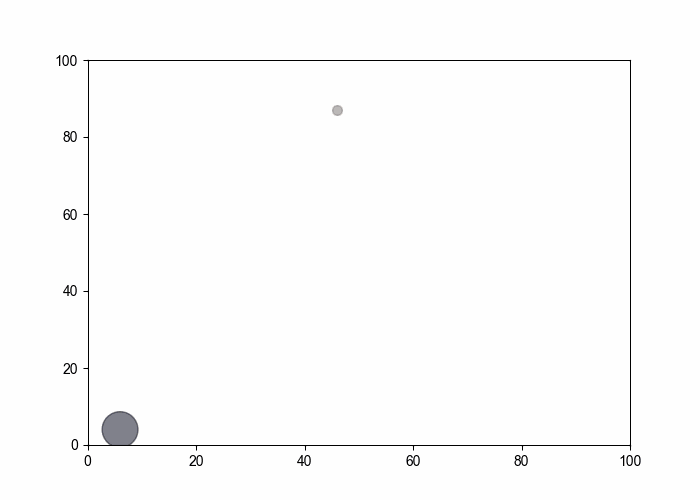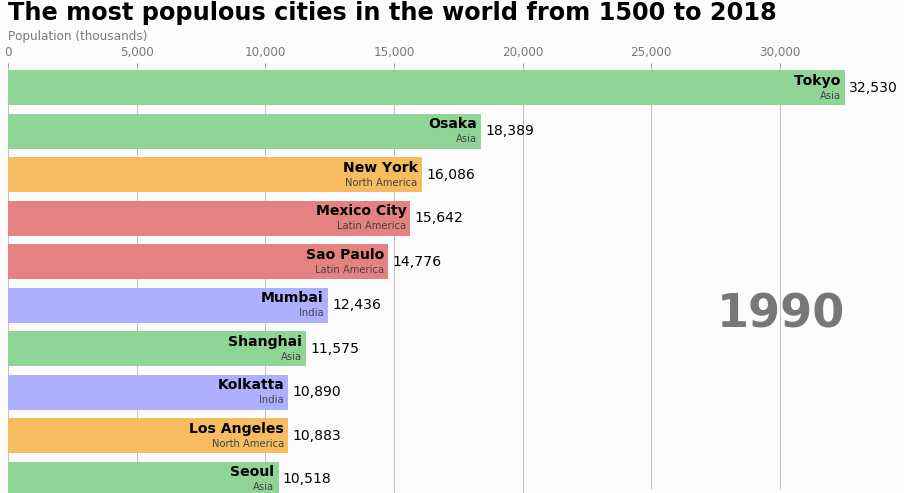# How to Create Animations in Python?

• Difficulty Level : Medium
• Last Updated : 14 Sep, 2021

Animations are a great way to make Visualizations more attractive and user appealing. It helps us to demonstrate Data Visualization in a Meaningful Way. Python helps us to create Create Animation Visualization using existing powerful Python libraries. Matplotlib is a very popular Data Visualisation Library and is used commonly used for graphical representation of data and also for animations using inbuilt functions.

There are two ways of Creating Animation using Matplotlib:

Attention geek! Strengthen your foundations with the Python Programming Foundation Course and learn the basics.

To begin with, your interview preparations Enhance your Data Structures concepts with the Python DS Course. And to begin with your Machine Learning Journey, join the Machine Learning - Basic Level Course

• Using pause() function
• Using FuncAnimation() function

## Method 1: Using pause() function

The pause() function in the pyplot module of the matplotlib library is used to pause for interval seconds mentioned in the argument. Consider the below example in which we will create a simple linear graph using matplotlib and show Animation in it:

• Create 2 arrays, X and Y, and store values from 1 to 100.
• Plot X and Y using plot() function.
• Add pause() function with suitable time interval
• Run the program and you’ll see the animation.

## Python

 `from` `matplotlib ``import` `pyplot as plt`` ` `x ``=` `[]``y ``=` `[]`` ` `for` `i ``in` `range``(``100``):``    ``x.append(i)``    ``y.append(i)`` ` `    ``# Mention x and y limits to define their range``    ``plt.xlim(``0``, ``100``)``    ``plt.ylim(``0``, ``100``)``     ` `    ``# Ploting graph``    ``plt.plot(x, y, color ``=` `'green'``)``    ``plt.pause(``0.01``)`` ` `plt.show()`

Output :Similarly, you can use the pause() function to create Animation in various plots.

## Method 2: Using FuncAnimation() function

This FuncAnimation() Function does not create the Animation on its own, but it creates Animation from series of Graphics that we pass.

Syntax: FuncAnimation(figure, animation_function, frames=None, init_func=None, fargs=None, save_count=None, *, cache_frame_data=True, **kwargs)

Now there are Multiple types of Animation you can make using the FuncAnimation function:

### Linear Graph Animation:

In this example, we are creating a simple linear graph that will show an animation of a Line. Similarly, using FuncAnimation, we can create many types of Animated Visual Representations. We just need to define our animation in a function and then pass it to FuncAnimation with suitable parameters.

## Python

 `from` `matplotlib ``import` `pyplot as plt``from` `matplotlib.animation ``import` `FuncAnimation``import` `numpy as np`` ` `x ``=` `[]``y ``=` `[]`` ` `figure, ax ``=` `plt.subplots()`` ` `# Setting limits for x and y axis``ax.set_xlim(``0``, ``100``)``ax.set_ylim(``0``, ``12``)`` ` `# Since plotting a single graph``line,  ``=` `ax.plot(``0``, ``0``) `` ` `def` `animation_function(i):``    ``x.append(i ``*` `15``)``    ``y.append(i)`` ` `    ``line.set_xdata(x)``    ``line.set_ydata(y)``    ``return` `line,`` ` `animation ``=` `FuncAnimation(figure,``                          ``func ``=` `animation_function,``                          ``frames ``=` `np.arange(``0``, ``10``, ``0.1``), ``                          ``interval ``=` `10``)``plt.show()`

Output:### Bar Plot Race Animation in Python

In this example, we are creating a simple Bar Chart animation that will show an animation of each bar.

## Python

 `from` `matplotlib ``import` `pyplot as plt``from` `matplotlib.animation ``import` `FuncAnimation, writers``import` `numpy as np`` ` `fig ``=` `plt.figure(figsize ``=` `(``7``,``5``))``axes ``=` `fig.add_subplot(``1``,``1``,``1``)``axes.set_ylim(``0``, ``300``)``palette ``=` `[``'blue'``, ``'red'``, ``'green'``, ``           ``'darkorange'``, ``'maroon'``, ``'black'``]`` ` `y1, y2, y3, y4, y5, y6 ``=` `[], [], [], [], [], []`` ` `def` `animation_function(i):``    ``y1 ``=` `i``    ``y2 ``=` `5` `*` `i``    ``y3 ``=` `3` `*` `i``    ``y4 ``=` `2` `*` `i``    ``y5 ``=` `6` `*` `i``    ``y6 ``=` `3` `*` `i`` ` `    ``plt.xlabel(``"Country"``)``    ``plt.ylabel(``"GDP of Country"``)``     ` `    ``plt.bar([``"India"``, ``"China"``, ``"Germany"``, ``             ``"USA"``, ``"Canada"``, ``"UK"``],``            ``[y1, y2, y3, y4, y5, y6],``            ``color ``=` `palette)`` ` `plt.title(``"Bar Chart Animation"``)`` ` `animation ``=` `FuncAnimation(fig, animation_function, ``                          ``interval ``=` `50``)``plt.show()`

Output:### Scatter Plot Animation  in Python:

In this example, we will Animate Scatter Plot in python using the random function. We will be Iterating through the animation_func and while iterating we will plot random values of the x and y-axis.

## Python

 `from` `matplotlib ``import` `pyplot as plt``from` `matplotlib.animation ``import` `FuncAnimation``import` `random``import` `numpy as np`` ` `x ``=` `[]``y ``=` `[]``colors ``=` `[]``fig ``=` `plt.figure(figsize``=``(``7``,``5``))`` ` `def` `animation_func(i):``    ``x.append(random.randint(``0``,``100``))``    ``y.append(random.randint(``0``,``100``))``    ``colors.append(np.random.rand(``1``))``    ``area ``=` `random.randint(``0``,``30``) ``*` `random.randint(``0``,``30``)``    ``plt.xlim(``0``,``100``)``    ``plt.ylim(``0``,``100``)``    ``plt.scatter(x, y, c ``=` `colors, s ``=` `area, alpha ``=` `0.5``)`` ` `animation ``=` `FuncAnimation(fig, animation_func, ``                          ``interval ``=` `100``)``plt.show()`

Output:### Horizontal Movement in Bar Chart Race:

• Here we will plot a Bar Chart Race using Highest Population in a city Dataset.
• Different cities will have a different bar and Bar Chart race will iterate from the year 1990 up to 2018.
• We selected countries from which the topmost cities would be picked from the dataset with the highest population.

 `import` `pandas as pd``import` `matplotlib.pyplot as plt``import` `matplotlib.ticker as ticker``from` `matplotlib.animation ``import` `FuncAnimation`` ` `df ``=` `pd.read_csv(``'city_populations.csv'``,``                 ``usecols``=``[``'name'``, ``'group'``, ``'year'``, ``'value'``])`` ` `colors ``=` `dict``(``zip``([``'India'``,``'Europe'``,``'Asia'``,``                   ``'Latin America'``,``'Middle East'``,``                   ``'North America'``,``'Africa'``],``                    ``[``'#adb0ff'``, ``'#ffb3ff'``, ``'#90d595'``,``                     ``'#e48381'``, ``'#aafbff'``, ``'#f7bb5f'``, ``                     ``'#eafb50'``]))`` ` `group_lk ``=` `df.set_index(``'name'``)[``'group'``].to_dict()`` ` `def` `draw_barchart(year):``    ``dff ``=` `df[df[``'year'``].eq(year)].sort_values(by``=``'value'``,``                                              ``ascending``=``True``).tail(``10``)``    ``ax.clear()``    ``ax.barh(dff[``'name'``], dff[``'value'``],``            ``color``=``[colors[group_lk[x]] ``for` `x ``in` `dff[``'name'``]])``    ``dx ``=` `dff[``'value'``].``max``() ``/` `200``     ` `    ``for` `i, (value, name) ``in` `enumerate``(``zip``(dff[``'value'``],``                                          ``dff[``'name'``])):``        ``ax.text(value``-``dx, i,     name,           ``                ``size``=``14``, weight``=``600``,``                ``ha``=``'right'``, va``=``'bottom'``)``        ``ax.text(value``-``dx, i``-``.``25``, group_lk[name],``                ``size``=``10``, color``=``'#444444'``, ``                ``ha``=``'right'``, va``=``'baseline'``)``        ``ax.text(value``+``dx, i,     f``'{value:,.0f}'``, ``                ``size``=``14``, ha``=``'left'``,  va``=``'center'``)``         ` `    ``# polished styles``    ``ax.text(``1``, ``0.4``, year, transform``=``ax.transAxes, ``            ``color``=``'#777777'``, size``=``46``, ha``=``'right'``,``            ``weight``=``800``)``    ``ax.text(``0``, ``1.06``, ``'Population (thousands)'``,``            ``transform``=``ax.transAxes, size``=``12``,``            ``color``=``'#777777'``)``     ` `    ``ax.xaxis.set_major_formatter(ticker.StrMethodFormatter(``'{x:,.0f}'``))``    ``ax.xaxis.set_ticks_position(``'top'``)``    ``ax.tick_params(axis``=``'x'``, colors``=``'#777777'``, labelsize``=``12``)``    ``ax.set_yticks([])``    ``ax.margins(``0``, ``0.01``)``    ``ax.grid(which``=``'major'``, axis``=``'x'``, linestyle``=``'-'``)``    ``ax.set_axisbelow(``True``)``    ``ax.text(``0``, ``1.12``, ``'The most populous cities in the world from 1500 to 2018'``,``            ``transform``=``ax.transAxes, size``=``24``, weight``=``600``, ha``=``'left'``)``     ` `    ``ax.text(``1``, ``0``, ``'by @pratapvardhan; credit @jburnmurdoch'``, ``            ``transform``=``ax.transAxes, ha``=``'right'``, color``=``'#777777'``, ``            ``bbox``=``dict``(facecolor``=``'white'``, alpha``=``0.8``, edgecolor``=``'white'``))``    ``plt.box(``False``)``    ``plt.show()`` ` `fig, ax ``=` `plt.subplots(figsize``=``(``15``, ``8``))``animator ``=` `FuncAnimation(fig, draw_barchart, ``                         ``frames ``=` `range``(``1990``, ``2019``))``plt.show()`